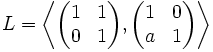# Dickson's theorem

## Statement

Suppose$F$ is a field of size$q$, where$q$ is a power of an odd prime$p$. Suppse$a$ is a generator of the multiplicative group of$F$. Consider the subgroup$L$ of the general linear group$GL(2,F)$ given by:$L = \left \langle \begin{pmatrix}1 & 1 \\ 0 & 1\\\end{pmatrix}, \begin{pmatrix}1 & 0\\a & 1\end{pmatrix}\right \rangle$.

Then, one of these cases holds:

•$L = SL(2,F)$, i.e.,$L$ is the entire special linear group.
•$q = 9$, and$L$ is a quasisimple group of order$120$, with$L/Z(L)$ isomorphic to the alternating group of degree five.

In either case,$L$ contains a subgroup isomorphic to SL(2,3).